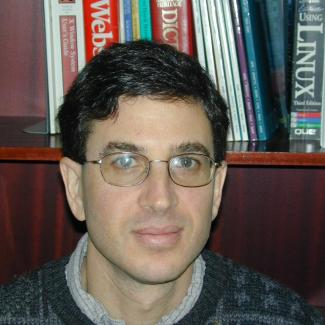Polynomial equations are ubiquitous in the mathematical sciences. The study of their solutions is the domain of algebraic geometry. Recently, there has been an explosion of activity, as computer scientists, physicists, applied mathematicians and engineers have realized the potential utility of modern algebraic geometry. This has brought forth an increased focus on quantitive and algorithmic questions. The semester will emphasize connections to geometric complexity theory. In this novel framework, fundamental lower bound questions can be rephrased and approached via representation theory and algebraic geometry. This applies to an arithmetic version of P versus NP as well as to multilinear algebra problems such as tensor rank and the complexity of matrix multiplication.

View the textbook written by J. M. Landsberg in connection with this program.

## OrganizersPeter Bürgisser (Technical University of Berlin; chair)Joseph M. Landsberg (Texas A&M University)Ketan Mulmuley (University of Chicago)Bernd Sturmfels (UC Berkeley)

## Long-Term Participants (including Organizers)

David Eisenbud (Mathematical Sciences Research Institute and UC Berkeley)
Wei Li (Chinese Academy of Sciences)
Ke Ye (University of Chicago)